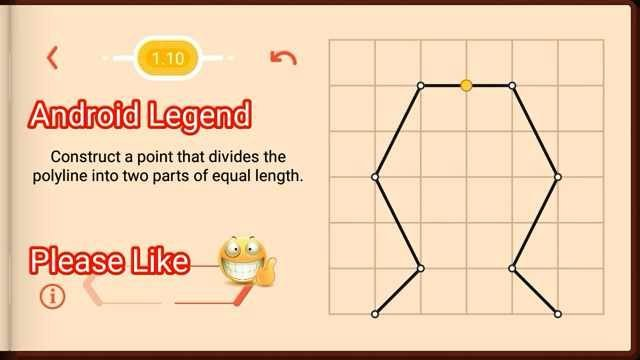Pythagorea Length and distance Level 1.10 Solution/AnswerPythagorea Length and distance Level 1.10 New Version Game Answers, Solutions, Tips, and Walkthroughs.

Pythagorea is android/iOS app developed by Horis International Limited. Solutions hints and answers to pythagorea are available in this post scroll down to find solutions to all the levels.

This game is mostly focused on geometric puzzles and construction. the workspace is divided into grids to draw lines. You should know all the basic Math operations. All lines and shapes are drawn on a grid whose cells are squares. Most of the game levels can be answered using natural intuition and by some basic laws of geometry.

Click Here for All other Pythagorea Levels:Pythagorea Length and Distance level 1.10: Construct a point that divides the poly line into two parts of equal length.If you have any doubt regarding Pythagorea answers given here you can watch video below or you can comment on this post-